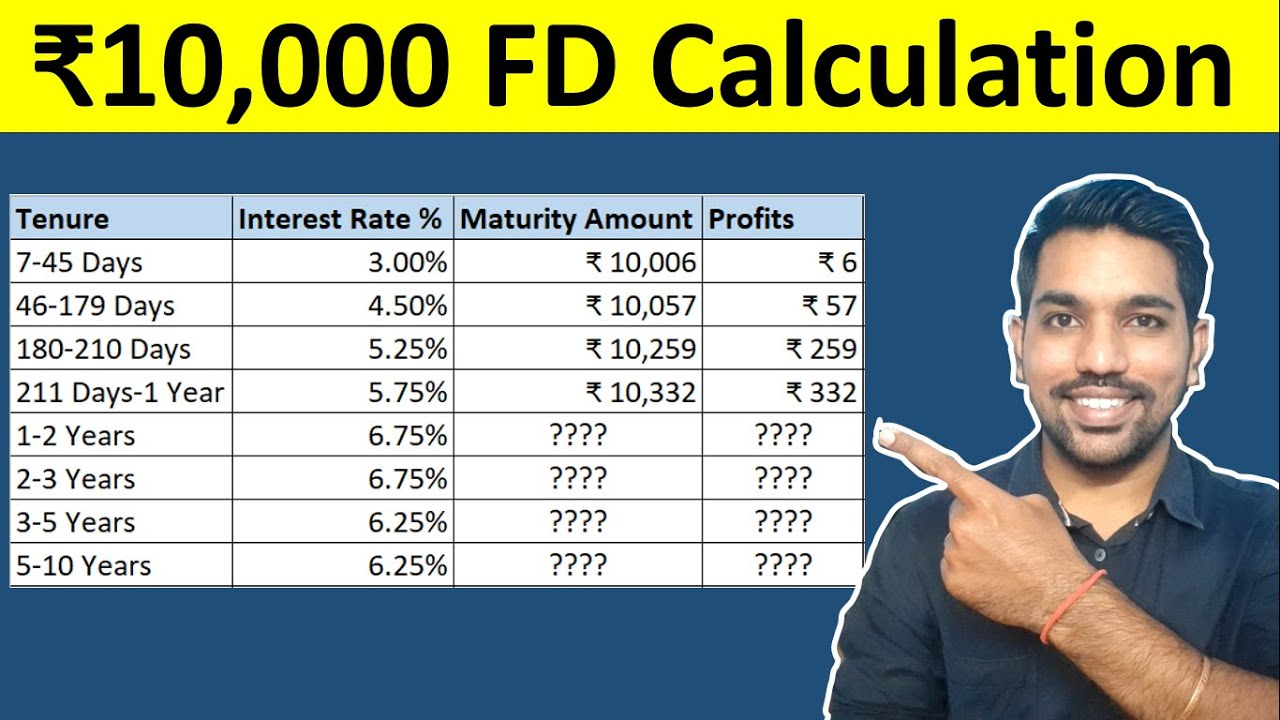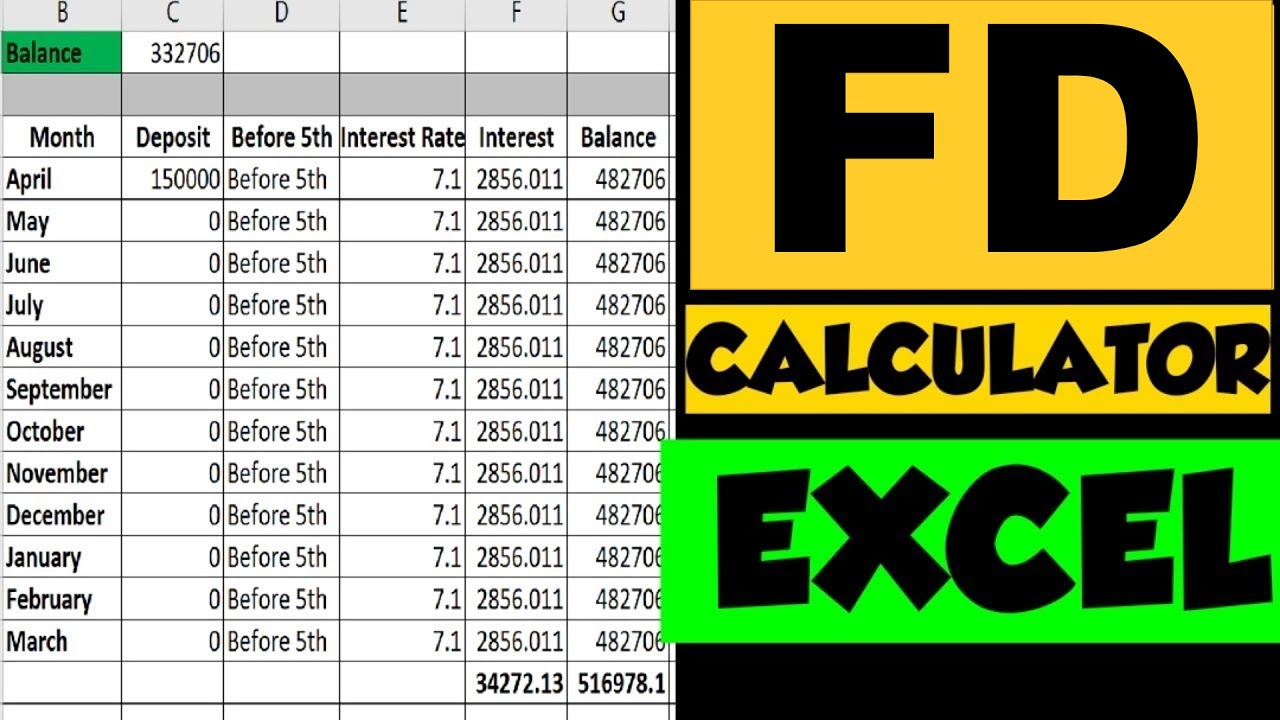# ₹10,000 FD Interest Calculation for Next 10 Years | Fixed DepositsHow is FD Interest Calculation done? How much interest you will get on FD of Rs. 10,000 from 7 days to 10 years? Is FD interest calculated monthly? How FD maturity is calculated? All these questions will be answered in this article.

FD or Fixed Deposits is a type of deposits in which we invest pre defined amount for a tenure with fixed interest rate. The FD interest rate is fixed throughout the tenure. FD interest is calculated on monthly basis using monthly interest rate and is compounded quarterly. Based on quarterly compounding and interest accumulation, maturity amount will be paid at the end of tenure.

Below is the video on how much Interest you will get in FD on Rs. 10000 deposit for next 5 year

## FD Interest Calculation on Rs. 10,000 for next 5 yearsWatch more Videos on YouTube Channel

As seen above, on Rs. 10,000 Fixed deposit for tenure of 5 years at interest rate of 6.25%, you will get total maturity of Rs. 13,635, for which total FD interest amount is Rs. 3,635.

## FD Interest Rates in SBI

The current interest rates in SBI (as of August 2023) are as follows:

As seen in above FD Interest Rates table, the FD interest rates for Senior citizens is 0.5% more as compared to non-senior citizens.

## IS FD INTEREST CALCULATED MONTHLY?

Yes, FD interest is calculated on monthly basis based on the monthly interest rate.

Moreover, FD interest is compounded on quarterly basis. Which means the interest calculated every month is added to the balance after every quarter which will earn more interest.

You can opt for non-cumulative Fixed Deposits as well, in which you receive FD interest every quarter instead of getting the interest compounded.

## How is FD Interest Calculated?

As mentioned above, FD interest is calculated on monthly basis based on the FD balance.

Below are the steps to calculated FD interest:

• Annual interest rate is divided by 12 to get monthly interest rate
• Monthly interest rate is than used to calculate monthly interest
• Monthly FD interest calculation formula is: monthly interest rate * balance / 100
• After every 3 months, accumulated monthly interest is added back to balance to earn more interest

Below is the example with Rs. 10,000 FD for 1 year at interest rate of 6.75%:

Let’s follow the steps to calculate FD Interest:

• Getting monthly interest rate – 6.75% / 12 = 0.5625%
• Calculating Monthly Interest on Balance of first month – 0.5625% * Rs. 10,000 / 100 = Rs. 56.25
• So we get first month interest as Rs. 56.25
• Similarly interest for all months are calculated.
• Note that once we have 3 months interest, total interest is added back to balance (For example: Month 4, Month 7, etc.)
• At the end, we get maturity amount of Rs. 10,692 after 1 year

We can also verify same using our online FD calculator here. Below is the screenshot from the FD calculator:

Note: You need to download the above excel calculator on computer or mobile and edit to calculate interest on your FD.

## How is FD payout calculated?

If you opt for non-cumulative FD, the interest accumulated every quarter will be paid out to you as quarterly interest.

In case of cumulative FD, the interest is accumulated every quarter and is added back to balance, on which you’ll get more interest in upcoming quarters.

So it depends on you, whether you want to take quarterly FD payout or total maturity amount at the end of tenure.

Below is the table for quarterly FD payout:

As seen above, you get total interest of Rs. 168.75 * 4 = Rs. 674 in non cumulative FD, compared to Rs. 692 in cumulative FD where you see compounding effect.

So compounding in FD helps us to gain more interest amount over long term.

ALSO READ: Latest Post Office Interest Rates

## FD Interest Calculation on Rs. 10,000

Let us now see how much interest you will get on Rs. 10,000 FD from 7 days to 10 years.

Below is the FD interest for all tenures with interest rates:

You can also watch below video on FD Interest Calculation using Excel:

### FD Interest Calculation in Excel VideoWatch more Excel Videos Here

## Conclusion

So FD is one of the best saving scheme which also helps in tax saving under section 80C (5 year tax saving FD). Cumulative FD will give more interest when it is compounded quarterly.

You can also receive quarterly payouts in which case your total interest will be less compared to compounding FD.

## You can Donate Too!

Found this Helpful? DONATE any amount to see more useful Content. Scan below QR code using any UPI App!

UPI ID: abhilashgupta8149-1@okhdfcbank

Verify that you are “Paying Abhilash Gupta” before making the transaction so that it reaches me. It makes my Day 🙂

Thank you for Donating. Stay Tuned!

## Income Tax Calculator App – FinCalC

For Income Tax Calculation on your mobile device, you can Download my Android App “FinCalC” which I have developed for you to make your income tax calculation easy.

### What you can do with this mobile App?

• Calculate Income Tax for new FY 2023-24 and previous FY 2022-23
• Enter estimated Investments to check income tax with Old and New Tax Regime
• Save income tax details and track regularly
• Know how much to invest more to save income tax
• More calculators including PPF, SIP returns, Savings account interest and lot more